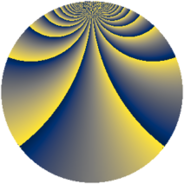# Properties

 Label 1045.2.bmLevel $1045$ Weight $2$ Character orbit 1045.bm Rep. character $\chi_{1045}(21,\cdot)$ Character field $\Q(\zeta_{18})$ Dimension $480$ Sturm bound $240$

# Related objects

## Defining parameters

 Level: $$N$$ $$=$$ $$1045 = 5 \cdot 11 \cdot 19$$ Weight: $$k$$ $$=$$ $$2$$ Character orbit: $$[\chi]$$ $$=$$ 1045.bm (of order $$18$$ and degree $$6$$) Character conductor: $$\operatorname{cond}(\chi)$$ $$=$$ $$209$$ Character field: $$\Q(\zeta_{18})$$ Sturm bound: $$240$$

## Dimensions

The following table gives the dimensions of various subspaces of $$M_{2}(1045, [\chi])$$.

Total New Old
Modular forms 744 480 264
Cusp forms 696 480 216
Eisenstein series 48 0 48

## Trace form

 $$480 q - 12 q^{3} - 36 q^{9} + O(q^{10})$$ $$480 q - 12 q^{3} - 36 q^{9} - 24 q^{14} + 24 q^{22} + 60 q^{26} + 36 q^{27} - 36 q^{31} - 144 q^{34} + 36 q^{36} + 24 q^{38} + 36 q^{42} - 12 q^{44} + 96 q^{47} - 204 q^{48} + 264 q^{49} + 72 q^{53} + 96 q^{58} - 108 q^{59} - 240 q^{64} - 126 q^{66} + 36 q^{67} + 108 q^{69} - 144 q^{70} + 12 q^{71} + 36 q^{77} + 300 q^{78} - 348 q^{81} + 36 q^{82} - 12 q^{86} - 144 q^{88} + 72 q^{89} - 24 q^{91} - 48 q^{93} - 60 q^{97} - 54 q^{99} + O(q^{100})$$

## Decomposition of $$S_{2}^{\mathrm{new}}(1045, [\chi])$$ into newform subspaces

The newforms in this space have not yet been added to the LMFDB.

## Decomposition of $$S_{2}^{\mathrm{old}}(1045, [\chi])$$ into lower level spaces

$$S_{2}^{\mathrm{old}}(1045, [\chi]) \cong$$ $$S_{2}^{\mathrm{new}}(209, [\chi])$$$$^{\oplus 2}$$